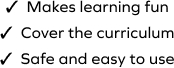# Subtract 2-Digit Number from 3-Digit Number

SplashLearn - The complete PreK - Grade 5 Math & ELA Learning Program Built for Your Child
Home > Subtract 2-Digit Number from 3-Digit Number
The game gives students plenty of opportunities to work with problems based on subtraction. They are encouraged to apply their mathematical knowledge to find the answer. It's important that students work at a level appropriate for them. This game requires learners to work with 3-digit and 2-digit numbers. Students will need to select the correct number to complete the tasks.